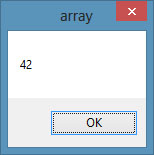# lesson 10 Creating Arrays

An array is a group of variables belongs to the same data type. When we deal with a single item, we only need to declare one variable. However, if we have a list of the similar type of data, it is better to declare an array of variables. For example, if we need to enter one thousand phone numbers, it would be very tedious if we were to declare one thousand different phone numbers. Therefore, instead of declaring one thousand different phone numbers, we need to declare only one array. We can differentiate each phone number in an array by using subscript, the index value of each item, for example, phnum(1), phnum(2),phnum(3) .......etc.

### 10.2 The Dimension of an Array

In VB2013,  arrays can be one dimensional or multidimensional. A one-dimensional array resembles a table that comprises one row of items or one column of items.

A multi-dimensional array is a table comprises rows and columns of items. The way to reference an element in a one-dimensional array is ArrayName(x), where x is the index or position number of the element. The way to reference an element in a two-dimensional array is ArrayName(x,y), where (x,y) is the index of the element. Usually, it is sufficient to use a one-dimensional and two-dimensional array, you only need to use higher dimensional arrays if you need to deal with more complex problems. Let's examine the arrays in the following tables.

 Customer Name Name(0) Name(1) Name(2) Name(3) Name(4) Name(5) Name(6)

 Name(0,0) Name(0,1) Name(0,2) Name(0,3) Name(1,0) Name(1,1) Name(1,2) Name(1,3) Name(2,0) Name(2,1) Name(2,2) Name(2,3) Name(3,0) Name(3,1) Name(3,2) Name(3,3)

### 10.2 Declaring an Array

We use Public or Dim statement to declare an array ,similar to the way we declare a single variable. The Public statement declares an array that can be used throughout an application while the Dim statement declares an array that can be used only in a local procedure .

The statement to declare a one-dimensional array is as follows:

`Dim arrayName(n) as dataType`

where n indicates the last index in the array. Note that n does not indicate the total number of elements in the array, it is one less than the total number of elements (n-1) because the first element is always the zeroth element. The first element is arrayName(0), the second element is arrayName(1), the third element is arrayName(2) and so on. The number of elements in an array is also known as length, we can retrieve the length of an array using the syntax arrayName.length

For example,

```Dim CusName(10) as String
```

declares an array that comprises 11 elements starting from CusName(0) through to CusName(10)

To determine the length of an array, you can write the following code:

#### Example 10.1

```Private Sub Form1_Load(sender As Object, e As EventArgs) Handles MyBase.Load
Dim CusName(10) As String
MsgBox(CusName.Length)
End Sub
```

Running the program produces a message box that displays the length of the array i.e. 11, as shown in Figure 10.1

We can also declare an array with a non-zero starting index by initializing an index value other than zero, as follows:

```Dim arrayname As DataType()
arrayName=New String(){1,2,3,....,n)
```

This array will consist of n elements, starting with arrayName(1)

#### Example 10.2

```Private Sub Form1_Load(sender As Object, e As EventArgs) Handles MyBase.Load
Dim CusName As String()
CusName = New String() {1, 2, 3}
MsgBox(CusName.Length)
End Sub
```

The message box displays the length as 3

The statement to declare a two-dimensional array is as follow:

`Dim ArrayName(m,n) as dataType`

where m and n indicate the last indices in the array. The length of the array is (m+1)x(n+1)

#### Example 10.3

```Private Sub Form1_Load(sender As Object, e As EventArgs) Handles MyBase.Load
Dim CusName(5,6) As String
MsgBox(CusName.Length)
End Sub
```
Running the program and the message box displays a length of 42, as shown in Figure 10.2Figure 10.2

#### Example 10.4

```Private Sub Form1_Load(sender As Object, e As EventArgs) Handles MyBase.Load
Dim num As Integer
Dim CusName(5) As String
For num = 0 To 5
CusName(num) = InputBox("Enter the customer name", "Enter Name")